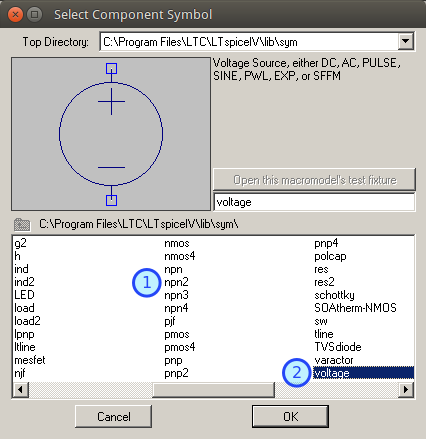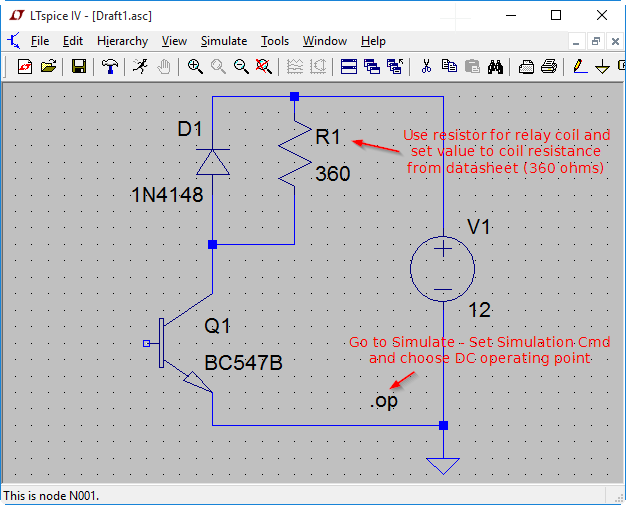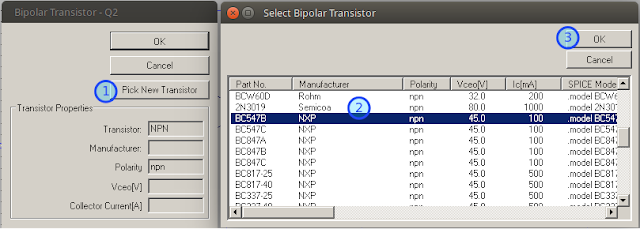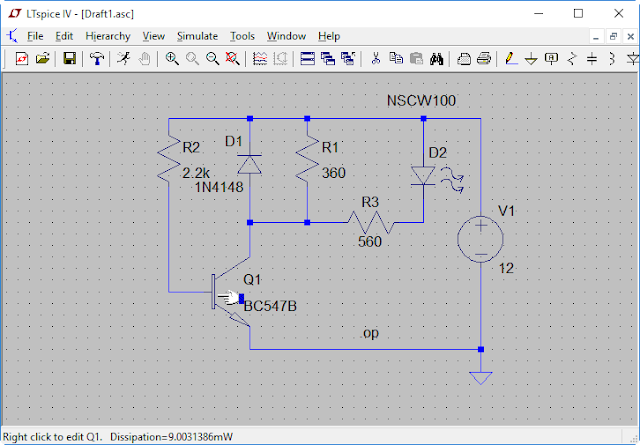### Free EDA: Design and simulation with LTspice

LTspice is Linear Technology's circuit simulation tool. It is free and very powerful. Learn to use it to design, simulate, analyze and measure electronic circuits.

This is the first part of a series articles about turning a circuit idea to design using only free software. EDA stands for electronic design automation and Wikipedia has a nice comparison table of software for this job.

Before drawing the schematic and designing the PCB we need a circuit idea. Then we will use the free circuit simulation software LTspice to simulate our design. This is what this article is about. LTspice is developed by Linear Technology and it runs native in Windows and Mac OS X. The Windows version runs without issues on Linux using Wine.

This is not a LTspice tutorial. For that we recommend Terry Sturtevant's and Simon Bramble's tutorials. You will also need electronics knowledge. See All About Circuits, Learn Electronics Online or Learn About Electronics.

For this article I'm gonna use a simple circuit idea: let's make a dark activated relay. This works by the following principle: a sensor measures light intensity and depending on what it reads turns on or off a relay. Because it is a dark activated relay, the relay will be triggered when light intensity drops below a set threshold. Here's what we need:
• light sensor: an LDR or photodiode (while searching for datasheets I already found example schematics for what I want to build);
• an opamp which we will use as voltage comparator;
• a driver transistor for the relay coil;
• a relay;
• some resistors, a LED, a diode.
The design is simple: we build a voltage divider using the LDR with a resistor and another one using fixed resistors. Then we use the opamp to compare the voltages from these dividers. Opamp will drive a transistor that will trigger the relay.

LTspice is easy to use. Just add the parts from the top toolbar can connect them with the wire tool. It is mandatory to add a ground symbol to every schematic. Don't forget to set values for each part otherwise simulation will not work. Then you go to Simulate menu and choose Edit simulation cmd. There are a few operation modes:
• DC operating point where all capacitors are treated as open circuit and all inductors as closed circuit in order to calculate quiescent voltage and currents for all parts and circuit nodes.
• Transient analysis calculates circuit behavior over a period of time.
• AC analysis sweeps an AC source parameters and calculates how the circuit behaves to that.
• DC sweep modifies chosen voltages, currents or other parameters.
Let's start drawing our circuit. I will draw it from right to left (from output to input). To do that, open LTspice and click the New Schematic (1) toolbar button (or the File menu entry). Now you have access to the main toolbar from where you can add basic components and perform various operations (cut, copy, paste, draw wires, move or drag symbols). We will use the Component (2) button to add a NPN transistor, a diode, a resistor and a voltage supply.LTspice main toolbar
When you click the Component icon you will be prompted with a library dialog. We will select npn2 for the transistor and voltage for the power supply. The entries between "[]" are folders that contain various components grouped by categories.LTspice component library
We will power it from 12 V so we need to choose a 12 V relay. G2RL series is very common and it draws only 33 mA (the general purpose type). We will place this relay in the collector of the driver transistor. We can use BC547 although its maximum collector current is rather low: 100 mA. Let's design this part of circuit and play a little with it. We are not interested in the inductive properties of relay's coil so we'll substitute it with a resistor. To protect the transistor we'll use a flyback diode (not really needed in the simulation).LTspice design
While you can easily right click most passive components and enter their value, for the semiconductors things are a little different. For example, if we right click the transistor symbol we will be prompted with a common transistors list that we can choose from. Those are the simulation models available by default in LTspice. BC547 is very common so we'll find it in the library.Set component type/value
Before running the simulation, let's turn on the transistor by biasing it. When on, the collector current will be 33 mA. Let's add an indicator LED of 15 mA parallel with the relay, so the collector current gets 33 + 15 = ~ 50 mA. The transistor hFE is at least 100. This means we can drive it on with at least 50 mA / 100 = 500 uA. A rule of thumb is to use 10 times greater current than this value, so 5 mA should be enough. We need to forward bias the BE junction of the transistor with 5 mA. The voltage drop across the junction is ~ 0.7 V and our power supply voltage is 12 V. So we will use a resistor that can drop 12 - 0.7 = 11.3 V at 5 mA. Using Ohm's law 11.3 / 0.005 = 2.2 kiloohms. Here is our new test schematic:LTspice DC operating point simulation
If you follow and added the DC operating point instruction, you can now run the simulation (Simulate - Run). A new window with voltages and currents will open. If you look at transistor collector and base currents you'll see they are as we wanted. After closing that window you can hover with the cursor on all parts and see important information. In the above screenshot, we can see the transistor power dissipation is 9 mW, which is great for a 500 mW rated transistor.

Let's add the opamp. We'll use LM393 for the real circuit and a generic opamp in LTspice (Opamps/UniversalOpamp2) and we'll connect the 2.2 k resistor to its output. We can reduce the resistor value (1.5...1.8 k) to compensate opamp voltage drops. Here is the completed schematic for simulation.Completed schematic
According to datasheet, the opamp can be driven by no less than 500 nA in worst case. So the voltage dividers should be able to supply at least 10 times 500 nA, thus 5 uA. Divide 12 V by 5 uA and get the maximum summed value of resistors that form the voltage divider: 2.4 megaohms. There is no need to get even close to that with our reference voltage divider, so anything from 10 to 100 k will do fine. The LDR (R6 in my schematic) resistance swings from 1 M at complete darkness to 400 ohms at 1000 lx accoring to datasheet. Again, this leaves us with plenty of choices for the other resistor in the divider (R7 in my schematic). The most important thing now is the trigger level. So we'll make use of the datasheet:Resistance vs. illumination
We want our relay to trigger at let's say 10 lx, so we will choose a 27...33 k resistor for R7. Notice in the screenshots that R6 has the value {R} because we use R6 to emulate the LDR. Therefore there is an additional SPICE command you can see there: .step param R 1k 1Meg 10k which tells LTspice to sweep value of R from 1k to 1M using 10k steps. I learned how to do that from here. Let's simulate:LTspice simulation
After running the simulation click any wire that connects to transistor collector (where the cursor turns into a probe). You'll get the green graph from my screenshot. That is the collector voltage. When it is 0 the relay is triggered. If you Alt+Click the transistor, you'll see the power dissipation of the transistor (blue graph).

That is it. You may wonder how can you turn this into a light activated relay. Method 1: switch opamp inputs or method 2: switch the LDR and its resistor (put the LDR to ground and the resistor to 12 V).

This was not intended to be an electronics lesson or a LTspice tutorial. This is just a demonstration of how you can use LTspice to design your circuits. It is assumed you already have electronics knowledge and know to use LTspice. Or at least now you want to learn.

The second part will show how you can draw beautiful schematics of your circuits. We will also add a preset to the reference divider so you can adjust trigger level.

Overall, this article described a simple circuit that can be easily designed and simulated using free tools. Do you use LTspice? If not what simulation software do you use to test your circuits?